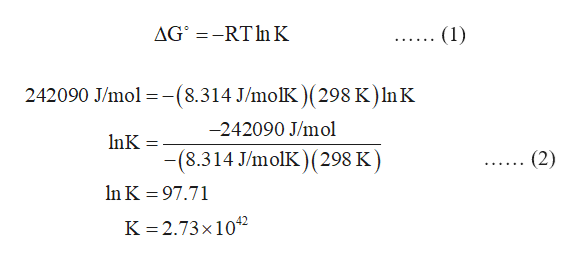# Acetylene, C2H2, can be converted to ethane, C2H6, by a process known as hydrogenation. The reaction isC2H2(g)+2H2(g)⇌C2H6(g)Given the following data at standard conditions (all pressures equal to 1 atm and the common reference temperature 298 K), what is the value of Kp for this reaction?SubstanceG∘f(kJ/mol)C2H2(g)209.2H2(g)0C2H6(g)−32.89

Question
1514 views

Acetylene, C2H2, can be converted to ethane, C2H6, by a process known as hydrogenation. The reaction is

C2H2(g)+2H2(g)⇌C2H6(g)

Given the following data at standard conditions (all pressures equal to 1 atm and the common reference temperature 298 K), what is the value of Kp for this reaction?

 Substance G∘f(kJ/mol) C2H2(g) 209.2 H2(g) 0 C2H6(g) −32.89
check_circle

Step 1

The given reaction is:

C2H2 (g) + 2H2 (g) ═ C2H6 (g)

The standard free energy change of the given reaction is calculated by subtracting the standard free energies of formation of reactants from that of the products.

Step 2

The conversion factor for kJ/mol and J/mol units is:

1 kJ/mol = 103 J/mol

Hence, -242.09 kJ/mol = -242.09 × 103 J/mol

= -242090 J/mol

Step 3

The relation between equilibrium constant and standard free energy change is given in the equation (1) in which ΔG0 is the standard free energy change, R is the universal gas const...help_outlineImage TranscriptioncloseAG RT n K .. (1) 242090 J/mol -(8.314 J/molK )(298 K ) In K -242090 J/mol InK -(8.314 J/molK) (298 K) (2) n K 97.71 K 2.73x 1042 fullscreen

### Want to see the full answer?

See Solution

#### Want to see this answer and more?

Solutions are written by subject experts who are available 24/7. Questions are typically answered within 1 hour.*

See Solution
*Response times may vary by subject and question.
Tagged in